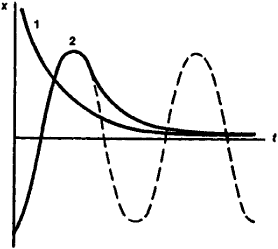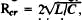Aperiodic System

Aperiodic System

a system in which natural oscillations are impossible because of excessive dissipation of energy. When an aperiodic system deviates from equilibrium position, the system slowly returns to that position (see Figure 1) and either never passes through the equilibrium position again, as in the case of curve 1, or passes through it only once and then returns to the equilibrium position, as in curve 2. This is not the case for oscillatory systems, which—as depicted in the dashed curve—return to the equilibrium position, passing it repeatedly.

An aperiodic system can be treated as the extreme case to which oscillatory systems approximate as energy losses in the systems increase. For example, an electrical oscillatory circuit becomes transformed into an aperiodic system when theFigure 1

electrical resistance R of the circuit, determining the energy losses in that circuit, attain the critical value, where C and L are respectively the capacitance and inductance of the circuit. Aperiodic systems are used in automatic control devices, broadband amplifiers of electrical oscillations, and so on.

Site: Follow: Share:
Open / Close